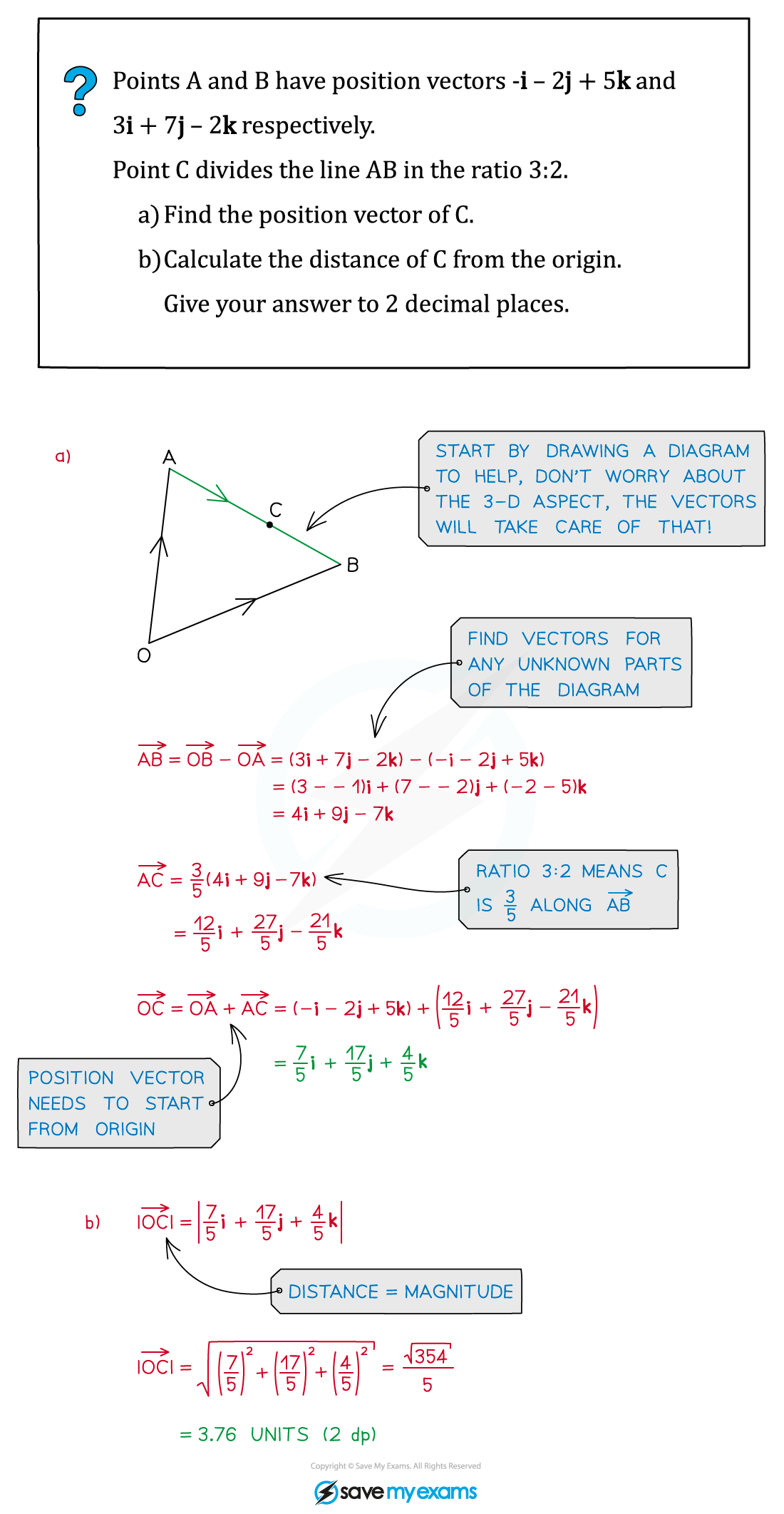# CIE A Level Maths: Pure 3复习笔记7.2.1 Vectors in 3 Dimensions

### Vectors in 3 Dimensions

#### What is a 3-D vector?

• Vectors represent a movement of a certain magnitude (size) in a given directionYou should have already come across (2D) vectors at AS (see Basic Vectors)
• 3-D vectors describe the position of a point in a 3-D space in relation to the origin
• They can be represented in different ways such as a column vector or in i, j, k unit vector form#### Magnitude of a 3-D vector

• The magnitude of a 3-D vector is simply its size
• Like 2-D vectors we can find the magnitude using Pythagoras’ theorem (see Magnitude Direction)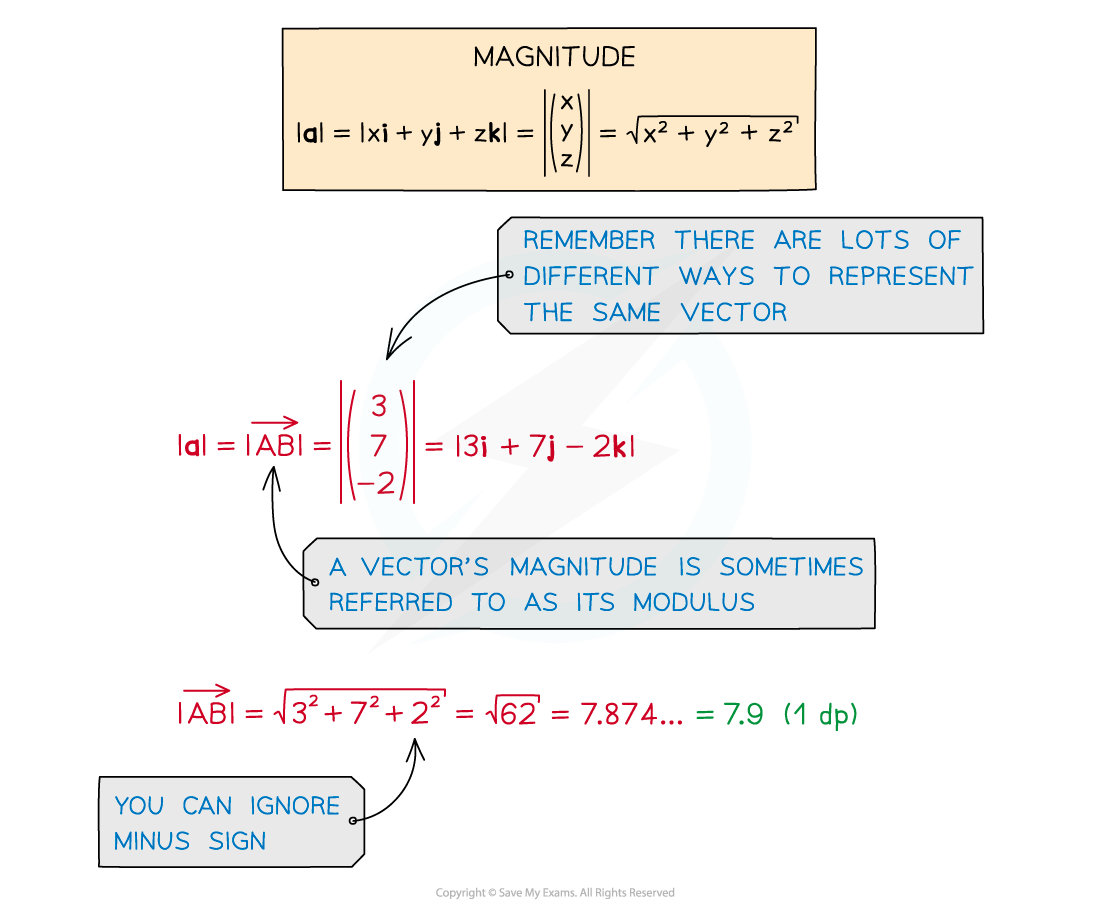• For 3-D position vectors we can find the distance between two points
• By using the respective co-ordinates we can calculate the magnitude of the vector between them: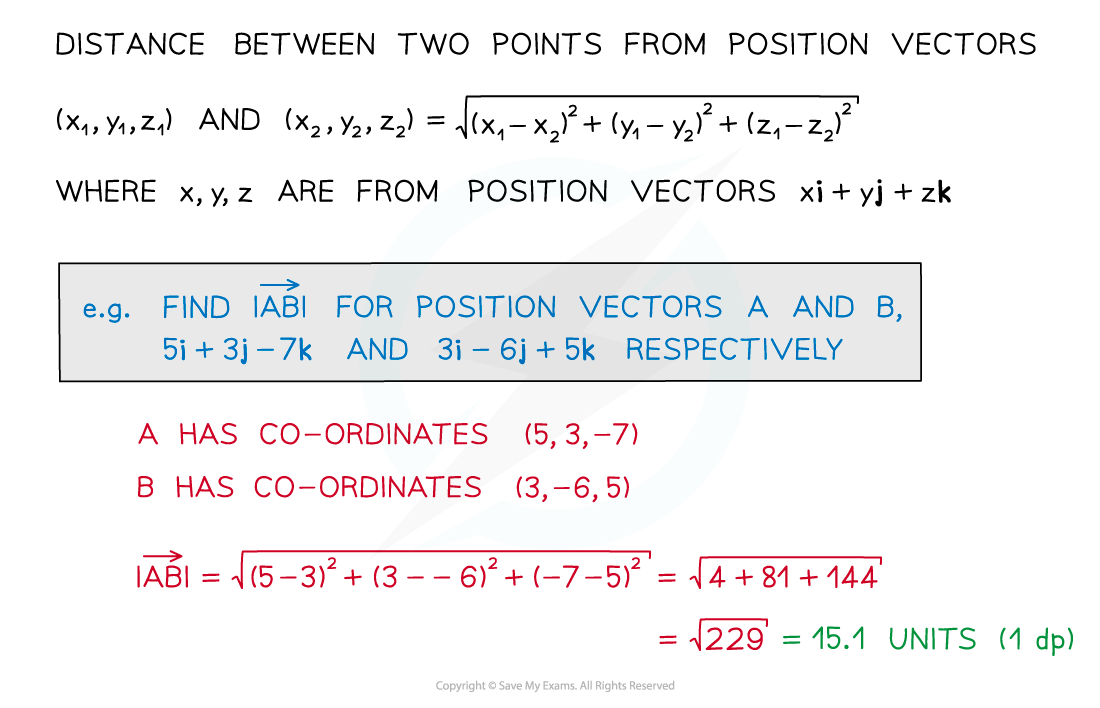#### 3-D vector addition, scalars, parallel vectors and unit vectors

• 3-D vectors work in the same way as 2-D vectors, just in three dimensions rather than two
• Vector addition and subtraction and scalar multiplication can be carried out in exactly the same way, this time involving i, j and k or x, y and z
• 3-D vectors are also parallel if one is a multiple of the other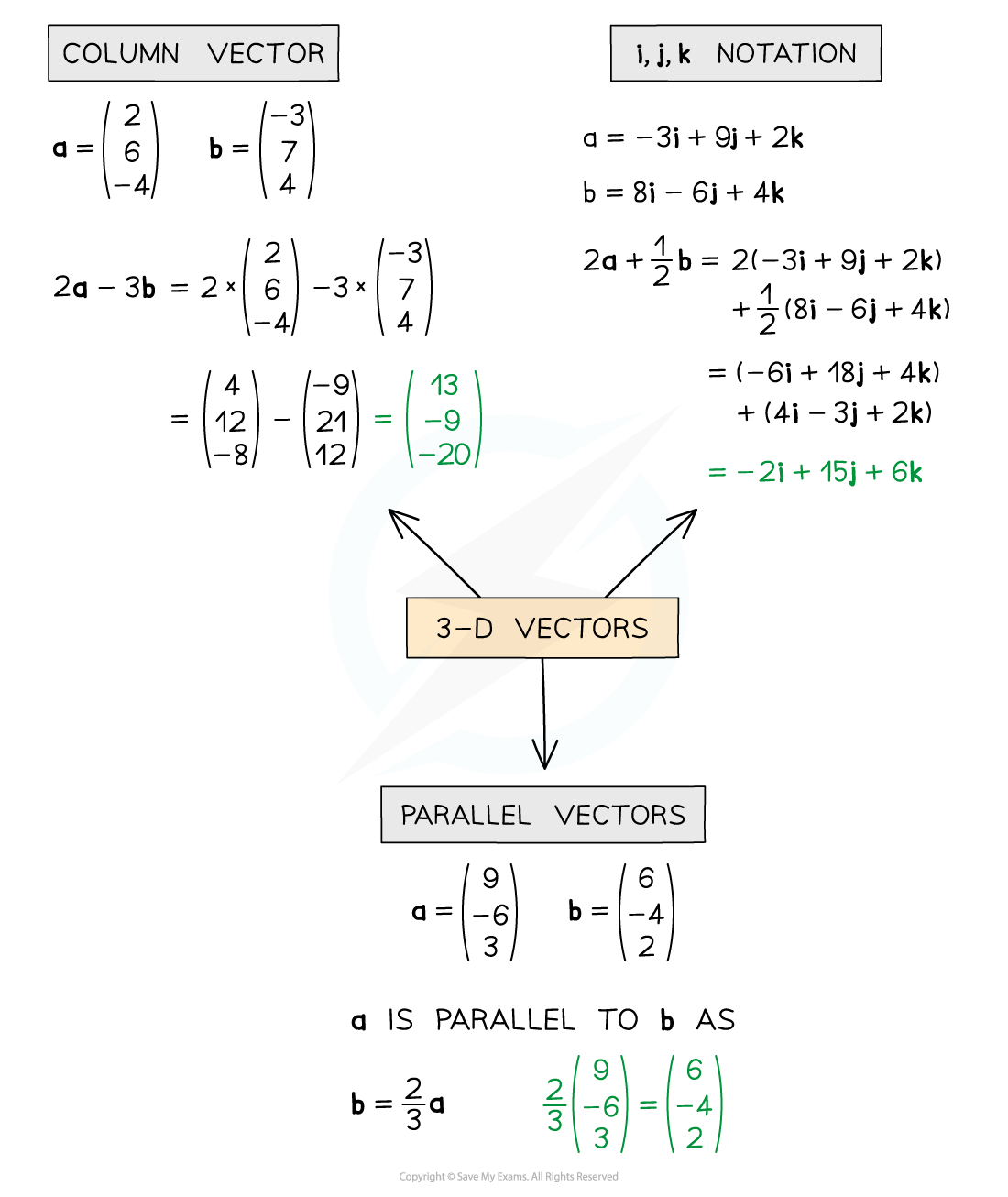• Unit vectors in 3-D are found in exactly the same way as in 2-D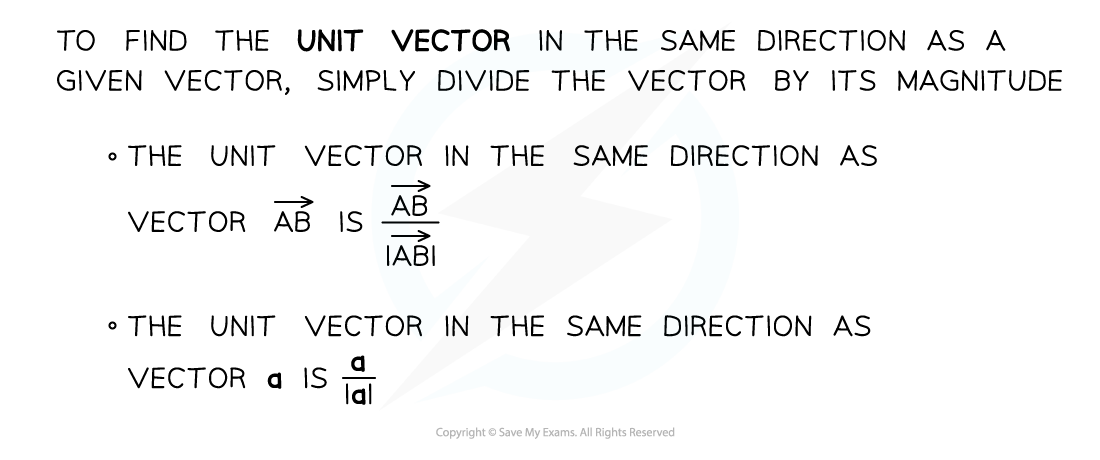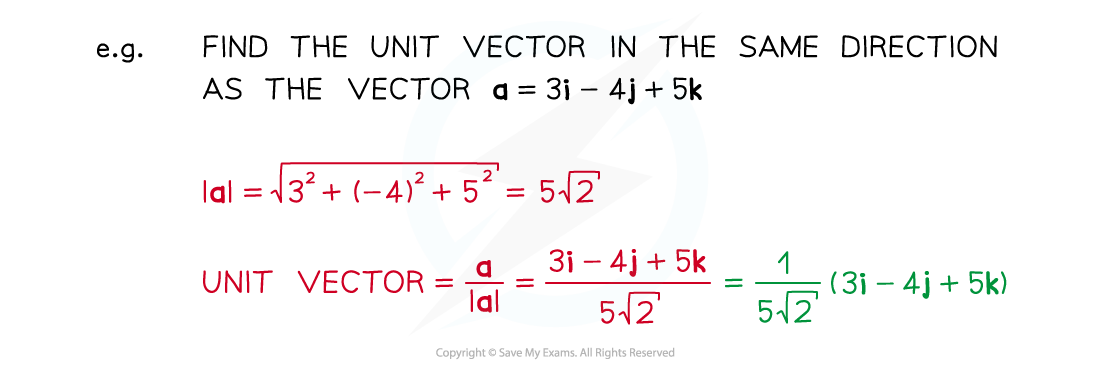#### Worked Example